# DAV Class 6 Maths Chapter 8 Brain Teasers Solutions

The DAV Class 6 Maths Solutions and DAV Class 6 Maths Chapter 8 Brain Teasers Solutions of Basic Geometrical Concepts offer comprehensive answers to textbook questions.

## DAV Class 6 Maths Ch 8 Brain Teasers Solutions

Question 1.
A. Tick (✓) the correct answer.
(a) A ________ extends endlessly in all directions.
(i) line
(ii) ray
(iii) plane
(iv) line segments
(iii) plane

(b) Which of the following is different from others?
(i) ball
(ii) orange
(iii) globe
(iv) sticker
(iv) sticker

(c) Three or more lines passing through the same point are called
(i) concurrent lines
(ii) intersecting lines
(iii) parallel lines
(iv) perpendicular lines
(i) concurrent lines

(d) The opposite side(s) of a classroom blackboard represent ________ line segments.
(i) vertical
(ii) parallel
(iii) intersecting
(iv) concurrent
(ii) parallel

(e) ________ line can pass through one given point.
(i) only one
(ii) only two
(iii) only three
(iv) many
(iv) many.(a) Give two examples of objects having curved surface.
Ball, globe.

(b) Four points A, B, C, D line on the same line l. What are these points called?
Collinear points.

(c) Can you draw a line on the surface of a sphere, completely lying on it?
No

(d) Give one example each of parallel and intersecting line segments from your environment.
Lines of copy; adjacent sides of black-board.

(e) If two lines m and n cut at a point X, what is the point X called?
Point of intersection.

Question 2.
You are given some points here. Name all the sets of collinear points.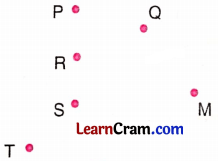P, R, S and Q, S, T are the two sets of collinear points.

Question 3.
In the figure given here, name:(a) all the pairs of parallel lines.
(b) 4 pairs of intersecting lines.
(c) the lines whose point of intersection is A.
(d) the lines whose point of inter section is D.
(e) 3 sets of collinear points.
(f) point of intersection of lines:
(i) p and r
(ii) l and p
(a) l ∥ m ∥ n
(b) p and r, p and m, p and Z, l and r
(c) q and l
(d) l and r
(e) (A, B, C), (D, E, F) and (H, I, J)
(f) (i) J
(ii) H

Question 4.
With the help of a figure, find the maximum and the minimum number of points of intersection of four lines in a plane.
In figure (i) A, B, C, D and E are the maximum number of points of intersection of 4 lines l, m, n and p.
∴ Total number of points is 4.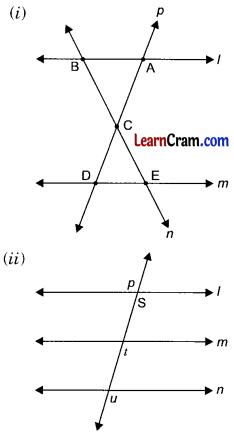In figure (ii) s, t, u are the minimum number of points of intersection of 4 lines 1, iii., n and p.
∴ The total number of points is 3.Question 5.
Write ‘True’ or ‘False’ for the following statements:
(a) Two lines in a plane always intersect at a point.
False

(b) Two different lines can be drawn passing through two given points.
False

(c) If two lines intersect at a point p, then p is called the point of intersection of the two lines.
True

(d) The minimum number of points of intersection of three lines is one. three lines which pass through the same point are called intersecting lines.
False

(e) Opposite sides of a blackboard represent parallel lines.
False

(g) A globe has a curved surface.
True

(h) Points which lie on the same line are called non-collinear points.
False

Question 6.
Fill in the blanks given below:
(a) The corner of a triangle represents a ________.
Point

(b) The table top gives an idea of a ________ surface.
Plane

(c) The ‘plus’ sign represents ________ line segments.
Intersecting

(d) A ________ extends infinitely in all directions.
Plane

(e) The lines of your notebook represent ________ lines.
Parallel

### DAV Class 6 Maths Chapter 8 HOTS

Question 1.
With the help of figures find the maximum number of points of intersection of four lines and five lines in a plane.
Do it yourself.

Question 2.
Look at the figure and answer the questions.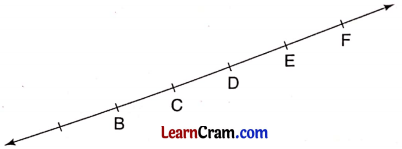(a) How many line segments are there in all?
(b) Name all line segments whose one end point is:
(i) E
(ii) C
(a) 15 line segments.
(i) EA, EB, EC, ED, EF
(ii) CA, CB, CD, CE, CF

### DAV Class 6 Maths Chapter 8 Enrichment Questions

Question 1.
Look at the figure and answer the questions.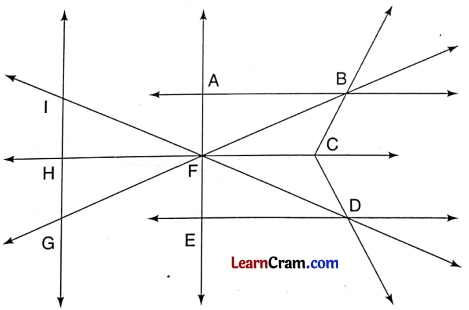(а) How many pairs of parallel lines are there?
5 pairs

(b) Name one set of four concurrent lines.
AE, ID, GB, HC

(c) Name the lines whose point of intersection is:
(i) A
(ii) H
(i) AB, AE
(ii) IG, HC

(d) Name the point of intersection of lines:
(i) ED and AE
(ii) IG and BG
(i) E
(ii) G

(e) Name two sets of three collinear points.
(I, G, H), (H, F, C)Question 1.
Classify the following figures as lines, rays and line segments.line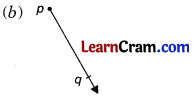raylineray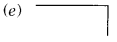line segmentsline

Question 2.
In the given figure state: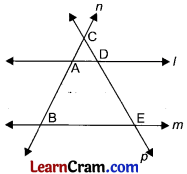(a) How many lines do you observe in the given figure?
(b) Name the parallel lines.
(c) Name the two sets of collinear points.
(d) Name all the intersecting lines.
(e) Name all the points of intersection.
(a) l, m, n and p represent the lines
(b) l ∥ m
(c) (C, D, E) and (C, A, B)
(d) l, m, p and n
(e) C, A, B, D and E

Question 3.
In the given figures, Name the points and line segments: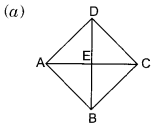Points are: A, B, C, D and E
Line segments: AB, BC, CD, DA, DB, AC, ED, EA, EB and EC.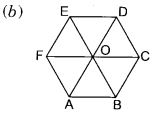Points are: A, B, C, D, E, F and O
Line segments: AB, BC, CD, DE, EF, FA, AD, BE, CF, OA, OB, OC, OD, OE and OF.Points are: A, B, C, D, E, F, G, O
Line segments: AB, BC, CD, DE, EF, FA, OA, OC, OE, BG, AF, CD, GD, FG.

Question 4.
In the given figure, find:(a) Number and name of lines.
(b) Sets of collinear points.
(c) Point of intersection of lines m and q.
(d) If l ∥ m ∥ n
(e) If $$\overline{\mathrm{AB}}=\overline{\mathrm{BC}}$$, then is it true for DE and EF?
(a) lines, l, m, n, p and q are the names of the 5 lines.
(b) (A, B, C) and (D, E, F)
(c) E
(d) Yes l ∥ m ∥ n
(e) Yes, it is true that DE = EFQuestion 5.
Fill in the blanks:
(a) Number of lines that can be drawn through two points is ________.
One

(b) ________ lines can be drawn through a point.
Infinite

(c) Two intersecting lines have one ________ point.
Common

(d) Parallel lines do not have ________ point.
Common

(e) Plane surface can be extended ________ is all directions.
Endlessly

(f) The lines intersecting at one point are called ________.
Concurrent

(g) A black board has ________ surface.
Plane

(h) The points which lie on the same line are called ________.
Collinear

Question 6.
Give two examples each of such letters of English alphabet having:
(a) Parallel lines
(b) Intersecting linesQuestion 7.
Give three examples each of the objects having:
(a) Flat surface
(b) Curved surface
(a) Black board, 100-rupee note, plane paper
(b) Ball, Orange, Egg

Question 8.
Lines p, q and r are concurrent. Also lines r, s and t are concurrent. Is it always true that the lines p, q and s will be concurrent? Is it also true for the lines p, q and f!(i) Yes, p, q and s will be concurrent.
(ii) Yes, it is true that p, q and t are also concurrent.

Question 9.
Can you draw a line on the surface of a sphere which lies wholly on it?
As the surface of the sphere is not plane.
So, we cannot draw any line on it.Question 10.
Give two examples from your environment of:
(a) Intersecting lines